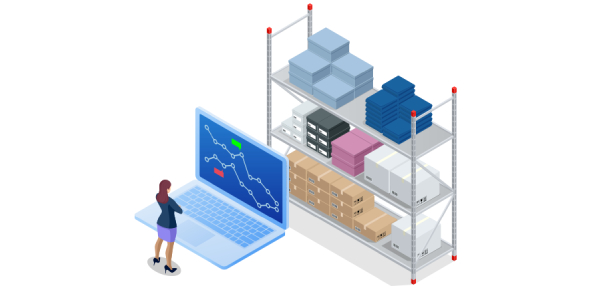# Inventory Management Quiz Questions

21 Questions | Total Attempts: 2976Settings.

• 1.
Materials management is defined as the
• A.

Management and control of inventory

• B.

management and control of services, inventory, and equipment

• C.

control of materials purchased

• D.

control of the amount of materials used for patient care

• 2.
Materials management can be classified in which two ways?
• A.

Equipment and materials

• B.

Ministration and clinical

• C.

• D.

Patient care and office materials

• 3.
Inventory management is the
• A.

Management and control of services, inventory, and equipment

• B.

management and control of inventory

• C.

control of supplies coming into the organization and supplies used

• D.

Control of materials purchased

• 4.
Why do managers sometimes have a hard time determining the right amount of supplies to have on hand?
• A.

The demand for supplies can fluctuate on the basis of patient volume.

• B.

Supplies may miss their delivery or be discontinued.

• C.

There is often a lag time between ordering and receiving the supply.

• D.

All of the above

• 5.
Where do costs related to inventory appear on an organization’s financial statements?
• A.

Current asset on the balance sheet

• B.

Expenses on the statement of revenues and expenses

• C.

Both of the above

• D.

None of the above

• 6.
Which of the following are methods for valuing inventory?
• A.

FIFO, LIFO, specific identification, weighted average

• B.

LIFO, FIFO, weighted average, frequent use

• C.

FO, LIFO, specific identification, frequent use

• D.

Specific identification, LIFO, FIFO, market method

• 7.
When using the LIFO method of valuing inventory
• A.

the last item put into inventory is the last item taken out

• B.

the last item put into inventory is the first item taken out

• C.

the last items taken out of inventory are items with no expiration date

• D.

Materials with a long shelf life are valued

• 8.
When using the FIFO method of valuing inventory
• A.

The first item taken out of inventory is the last item taken out

• B.

the first items taken out of inventory have a short shelf life

• C.

The first item put into inventory is the first item taken out

• D.

Materials with a short shelf life are valued

• 9.
Carrying costs
• A.

are the costs associated with holding an inventory of items

• B.

include a holding cost

• C.

are the costs associated with having vendors hold supplies for organizations

• D.

(a) and (b)

• 10.
What are costs associated with having more than enough inventory in stock called?
• A.

Stock-out costs

• B.

Holding costs

• C.

Overstock costs

• D.

Carrying costs

• 11.
What are costs associated with having too little inventory in stock called?
• A.

Stock-out costs

• B.

Opportunity costs

• C.

Overstock costs

• D.

Carrying costs

• 12.
Economic order quantity establishes
• A.

The maximum number of items an organization would want to purchase at one time

• B.

the quantity of items an organization should order each time to minimize costs associated with ordering

• C.

the number of items an organization can order in bulk to receive a discount

• D.

the quantity of items an organization would have to order each time to maximize costs associated with ordering

• 13.
The inventory turnover ratio measures
• A.

how quickly an organization goes through routine supplies

• B.

Inventory expenses in relation to operating revenue

• C.

costs incurred from ordering supplies in relation to total inventory expense

• D.

The number of times inventory is turned in relation to operating revenue

• 14.
Using the Economic Order Quantity formula and the Total Cost formula and given the following data, what is the EOQ? Price = \$100 Demand = 1,000 Ordering cost = \$10 Interest = 5% Holding cost = \$.50 Lag time = 5 days
• A.

48 units

• B.

58 units

• C.

68 units

• D.

78 units

• 15.
Using the data provided above, what is the Total Cost?
• A.

\$100,346

• B.

90,028

• C.

108,028

• D.

\$118,028

• 16.
Using the data provided above and given a constant demand, how many orders are placed each year?
• A.

15

• B.

17

• C.

19

• D.

21

• 17.
Using the data provided above and given a constant demand and a lag time of 5 days, how many units remain on the shelf when an order is placed?
• A.

10

• B.

12

• C.

14

• D.

16

• 18.
Using the data provided above and given a constant demand, how many weeks go by between orders?
• A.

One

• B.

Two

• C.

three

• D.

Four

• 19.
Time as a consideration is unimportant in inventory management.
• A.

True

• B.

False

• 20.
Just-in-time inventory is a method of holding inventory in the organization so that it can be accessed immediately prior to use.
• A.

True

• B.

False

• 21.
I worked the following Practice Problems
• A.

Inventory valuation, pgs 252-253

• B.

Economic order quantity, pgs. 255-256.

• C.

neither a or b

• D.

A and B

Related TopicsBack to top